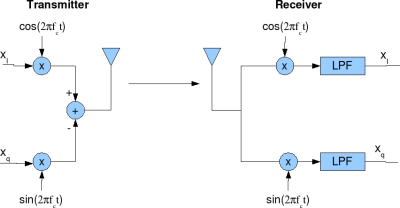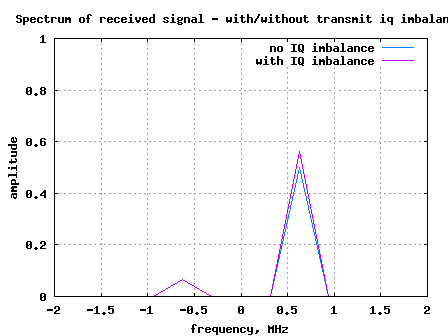- DSP log - http://www.dsplog.com -

IQ imbalance in transmitter

Posted By Krishna Sankar On March 8, 2009 @ 7:31 pm In Analog | 26 Comments

Typical communication systems use I-Q modulation and we had discussed the need for I-Q modulation  in the past. In this post, let us understand I-Q imbalance and its effect on transmit signal.

## I-Q modulation and demodulation

The typical I-Q modulation and demodulation is as shown in the figure below. Consider that the information to be transmitted is a complex signal,

.

At the output of I-Q modulation transmitter is,Figure: I-Q modulation Transmitter and receiver

At the transmitter, the information is sent on and is sent on .

At the receiver, we multiply the signal with and followed by low pass filtering (LPF) to extract, and respectively.

From trigonmetric identies,
,

,

.

The math for extracting the information at the receiver is as follows:

.

Similarly for the Q-arm,

.

Ignoring the scaling factor of 1/2, we are able to recover both and .

## Phase imbalance in IQ modulation

In an ideal I-Q modulator, the phase difference between the signals used for modulating the I arm and Q arm is 90degrees, resulting in using and for sending and .

When there is phase imbalance, the phase difference might not be exactly 90 degrees. We can consider that is used for sending and for sending .

## Amplitude imbalance in IQ modulation

When there is amplitude imbalance, there is small variation in the amplitude of thse sine and cosine arms in the modulator. This can be modelled as using for sending and using for sending , where is constant lying between 0 and 1.

The transmit signal including the effect of phase and amplitude imbalance is,

.

## Received signal with IQ imbalance in transmitter

Assuming that we have ideal IQ demodulator ie. at the receiver, we multiply the signal with and followed by low pass filtering (LPF) to extract, and respectively.

.

.

As can be observed from the above equations, the desired signal is distorted due to the presence of IQ imbalance.

## Effect on spectrum due to I-Q imbalance

To understand the effect of I-Q imbalance on the transmit signal, let us consider that the transmitted signal is i.e,

and

From the post on negative frequency , we know that such signal has a frequency component at and NO frequency component at .

In the presence of I-Q imbalance at the transmitter, the received signal is,

and

.Figure: Spectrum of received signal in the presence of IQ imbalance at the transmitter

It is reasonably intuitive to see that the received signal has frequency components at and also at . The component at was introduced due to I-Q imbalance.

Update : Click here for the derivation of Image Rejection Ratio (IMRR) with transmit IQ gain/phase imbalance 

URL to article: http://www.dsplog.com/2009/03/08/iq-imbalance-in-transmitter/

URLs in this post:

 need for I-Q modulation: http://www.dsplog.com/2008/04/05/need-for-i-q-modulator-and-demodulator/

 negative frequency: http://www.dsplog.com/2008/08/08/negative-frequency/

 Matlab/Octave script for plotting receive spectrum with transmit IQ imbalance: http://www.dsplog.com/db-install/wp-content/uploads/2009/03/script_transmit_iq_imbalance.m

 here for the derivation of Image Rejection Ratio (IMRR) with transmit IQ gain/phase imbalance: http://www.dsplog.com/2013/01/31/imrr-transmit-iq-gain-phase-imbalance/